﻿ Geometric Optimization of a Tuning Fork in NX Nastran

### Geometric Optimization of a Tuning Fork in NX Nastran

American Journal of Mechanical Engineering

## Geometric Optimization of a Tuning Fork in NX Nastran

Róbert Huňady1,, Peter Pavelka1

1Department of Applied Mechanics and Mechanical Engineering, Technical University of Košice, Faculty of Mechanical Engineering, Košice, Slovakia

### Abstract

A tuning fork is a simple tool used by musicians to tune their instruments or by medical practitioners to assess a patient's hearing. It produces a very pure and fixed tone, with most of the vibrational energy at the fundamental frequency, and little at its harmonic frequencies. To achieve a required tone it is needed to determine the exact dimensions of a tuning fork with regard to the material used for its manufacturing. Geometric optimization is an ideal design tool for this purpose. Paper deals with the process of proposal and design optimization of a common tuning fork by using NX Nastran software. Paper is divided into two parts. The first part deals with the experimental and numerical modal analysis of an existing tuning fork with the prongs of circular section. The second part describes the proposal and optimization of a new tuning fork with the prongs of square section.

• Róbert Huňady, Peter Pavelka. Geometric Optimization of a Tuning Fork in NX Nastran. American Journal of Mechanical Engineering. Vol. 4, No. 7, 2016, pp 320-324. http://pubs.sciepub.com/ajme/4/7/16
• Huňady, Róbert, and Peter Pavelka. "Geometric Optimization of a Tuning Fork in NX Nastran." American Journal of Mechanical Engineering 4.7 (2016): 320-324.
• Huňady, R. , & Pavelka, P. (2016). Geometric Optimization of a Tuning Fork in NX Nastran. American Journal of Mechanical Engineering, 4(7), 320-324.
• Huňady, Róbert, and Peter Pavelka. "Geometric Optimization of a Tuning Fork in NX Nastran." American Journal of Mechanical Engineering 4, no. 7 (2016): 320-324.

 Import into BibTeX Import into EndNote Import into RefMan Import into RefWorks

1234
Prev Next

### 1. Introduction

Computer Aided Engineering (CAE) is a field that has grown rapidly the last decades and has become essential in the field of engineering activities. A wide range of different design and analysis tools help to streamline the product development process. During the structural design process, the best decisions are made with respect to different aspects like stiffness, strength, construct ability and aesthetic property .

In structural optimization, use of different sets of data representing a mathematical model describes the behavior of a structure. Different control parameters are tuned by a set of design variables to find a situation in which the structure meets a given property . It is common to divide structural optimization into the following three types:

• Geometric optimization involves different size parameters of the structure that is to be optimized. It is common to relate this kind of optimization to a problem where you have a truss containing beams, and you change the thickness of each beam. 

• Shape optimization is where you optimize a structure by changing contour or form without changing topology. Shape optimization has an interdisciplinary character, meaning it can be used on a wide arrange of problems. This kind of optimization is more complex than the sizing optimization. It involves mathematical disciplines as partial differential equations, approximations of these and theory of nonlinear mathematical programming. In terms of three dimensional models and finite element methods, advanced software is required. [3, 4]

• Topology optimization optimizes the topology by, for example, making holes in the component. The algorithm changes the density of elements, controlling the stiffness contribution from that particular element. The result from the optimization must be interpreted and smoothed by the engineer, as output geometries are highly organic shapes that must be processed before production. Today, gradient-based algorithms are mostly implemented in commercial software, however new algorithms are continuously developed. For a more detailed description, see .

In this paper, the main attention is devoted to the geometric optimization, task of which is to modify the dimensions of the CAD model geometry to achieve required structural properties.

### 2. Theoretical Background of Geometric Optimization

The first step in the geometric optimization is to define a design model. This model is important for optimization process, the same as an analysis model for finite element analysis. The design model represents a formal statement of allowable changes that might be made to the structure during the search for an optimal design. It also places limits on these allowable changes, and defines limits on the structural responses. The design model usually contains the definitions of:

• design variables,

• design objective,

• design constraints.

The mathematical region over which design variables, objective, and constraints are defined is called the design space. The design variables are variables (geometric dimensions, parameters, etc.) that may be modified. There should be also described the relationship between the design variables and the analysis model properties. The design objective is represented by a function, which provides a scalar measure of design quality. The design constraints limit the design responses to an acceptable range.

The design optimization task can be mathematically expressed as follows :

• minimize (maximize) the objective function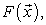(1)

• subject to inequality constraints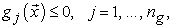(2)

• equality constraints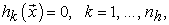(3)

• and side constraints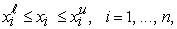(4)

where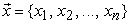are the design variables.

As can be seen, the objective function is a function of the design variables. It is the scalar quantity, that may either be linear or nonlinear, the same as the constraint functions. Although the problem is usually stated as a minimization task, it is possible maximize the objective function by minimizing its negative.

The searching for an optimal solution requires the use of an appropriate gradient-based algorithm that for a given point in the design space determines the gradients of the objective function and constraints, and then proceeds in this direction as far as it is possible to go. The process repeats until no further improvement in the objective cannot be made without violating any of the constraints. Since, the gradient vector is oriented in the direction of increasing objective function, to minimize it, it is necessary to move in an opposite direction. Therefore, the search vector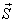is defined by the negative of the objective function gradient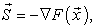(5)

where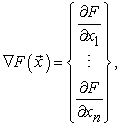(6)

where each partial derivative is a single component of the dimensional vector.

In the case of so called one-dimensional search, when huge number of design variables can be updated by varying the single parameter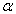, the new design at the conclusion of the search in the given directioncan be written as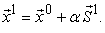(7)

The new objective and constraints can now be expressed as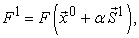(8)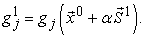(9)

From this new point in the design space, we can again compute the gradients and establish another search direction based on this information. Again, we will proceed in this new direction until no further improvement can be made, repeating the process, if necessary.

The numerical search algorithm requires to have a formal definition of an optimum. Any trial design can then be measured against this criteria to see if the optimum has been found. This required definition is provided by the Kuhn-Tucker conditions. Figure 1 shows an example of a two design variable space with constraints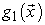and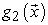and objective function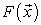. The optimum point in this example is the point that lies at the intersection of the two constraints.Download asVeiw figureFigures index
Figure 1. Kuhn-Tucker condition at a constrained optimum 

For this situation, when all the gradients point off in different directions, the Kuhn-Tucker conditions state that the vector sum of the objective and all active constraints must be equal to zero given an appropriate choice of so called Lagrange multipliers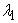,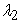. Figure 2 shows howandenable the zero vector sum condition to be met. The Kuhn-Tucker conditions are useful even if there are no active constraints at the optimum. In this case, only the objective function gradient is considered, and this is identically equal to zero; i.e., any finite move in any direction will not decrease the objective function .Download asVeiw figureFigures index
Figure 2. Graphical interpretation of Kuhn-Tucker conditions 

### 3. Modal Analysis of a Tuning Fork of Circular Section

In this section, the modal parameters of a common tuning fork of circular section are investigated by using numerical and experimental approach. The analyzed tuning fork has been made of steel and produced the tone A4 with the fundamental frequency of 440 Hz. Its shape and dimensions are obvious from Figure 3a.Download asVeiw figureFigures index
Figure 3. The tuning fork: a) dimensions, b) FEM model, c) experimental model
3.1. Numerical Modal Analysis

The numerical modal analysis was performed in NX Nastran (SOL 103) . A FEM model of the tuning fork is shown in Figure 3b. The geometry was divided into the simpler bodies to achieve an appropriate finite element mesh. The mesh consisted of 3D elements Tetra 10. The material properties corresponds to steel with Young's modulus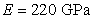and density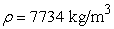. The Lanzos eigenvalue method was used to determine natural frequencies and mode shapes. No constraints were considered at the analysis. Therefore, a lower frequency range limit of the solution was set to 1 Hz to exclude rigid-body modes. The upper limit was 3200 Hz.Download asVeiw figureFigures index
Figure 4. The mode shapes and natural frequencies of the tuning fork obtained by numerical analysis in NX Nastran

The 5 flexible modes were identified in the given frequency range. Their vibration shapes and natural frequency values are shown in Figure 4.

3.2. Experimental Modal Analysis

In the second phase, the modal parameters of the tuning fork were determined experimentally by using the measurement system PulseTM produced by Bruel&Kjaer company. With respect to the dimensions of the measurement object, the one-point laser Doppler vibrometer Polytec PDV-100 had to be used to measure the vibration responses. The object was excited by the impact hammer Bruel&Kjaer 8206 with a plastic tip. The experimental model contained one input and six output degrees-of-freedom. Due to geometrical symmetry, only half the model was considered. The responses were only measured in the direction that is essential in term of the tuning fork functionality. The experimental model is schematically depicted in Figure 3b.

In practice, free support is usually realized either by putting the object on a very soft pad (e.g. foam) or suspending it on soft springs. It is obvious that frequencies of rigid body modes will not be equal to zero in that case, but the values will be very low. Such support is considered to be free if the highest natural frequency of rigid body modes is less than 10% of the value of the lowest deformation natural frequency . In our case, the spherical end of the tuning fork was covered by a piece of polyurethane foam and softly clamped between the jaws as can be seen in Figure 5. This way of support satisfied the above condition.Download asVeiw figureFigures index
Figure 5. The measurement setupDownload asVeiw figureFigures index
Figure 6. Complex Mode Indicator Function plot

The frequency range of the measurement was from 0 to 3200 Hz. Figure 6 shows the CMIF plot in which four dominant peaks can be identified in this range.

The first peak with the frequency of 12.377 Hz relates to a translation rigid-body mode. All the other peaks belong to the modes of the tuning fork. Their frequency and mode shapes are shown in Figure 7.Download asVeiw figureFigures index
Figure 7. The mode shapes and natural frequencies of the tuning fork obtained by experimental modal analysis

The natural frequency values obtained by the measurement are in good agreement with the frequencies determined by FEM analysis. The natural frequency of the second mode is shifted to higher value due to added stiffness in the region of the constraint.

### 4. The proposal and geometric Optimization of a New Tuning Fork

The proposal was based on the following requirements:

• material: steel,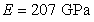,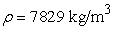,

• fundamental frequency: 440 Hz,

• square section of prongs.

An initial design and dimensions of the proposed tuning fork is shown in the Figure 8a.Download asVeiw figureFigures index
Figure 8. a) The initial design and dimensions of the proposed tuning fork. b) FEM model

In the first step, the numerical modal analysis was carried out to determine the natural frequencies and mode shapes of the tuning fork. The conditions of the simulation were the same as in the previous case. For the initial FEM model shown in Figure 8b, the frequency of the first mode was 693.55 Hz.

In the second step, the geometric optimization was performed to obtain dimensions corresponding to the required properties. In NX Nastran, geometry optimization is performed by an NX Optimizer tool that is based on software called Altair HyperOpt. The Altair hyperOpt is an adaptive response surface method (ARSM). Hyperopt uses a quadratic polynomial that is found and updated for each of the iterations. These are based on current and previous iterations. NX Optimizer can realize only single-objective optimization; i.e. that only one objective is considered. It can either be to maximize, minimize or reach a certain target for the objective function by changing the assigned parameters. In our case, value of the first natural frequency was used as the objective function. Its target value was set to 440 Hz. The thickness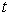and length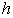of the prongs were used as design variables with following limits: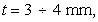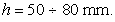No design constraints were defined.

The optimization process was done within 5 design cycles. The results from the individual cycles are listed in Table 1. Figure 9 graphically describes the changes of the design variables and objective function.

#### Table 1. The optimization process historyDownload asPowerPoint Slide

Veiw figure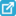View current table in a new windowDownload asVeiw figureFigures index
Figure 9. The optimization process history

The final design of the tuning fork with optimized dimensions is shown in Figure 10, as well as its mode shapes and natural frequencies.Download asVeiw figureFigures index
Figure 10. The final design and mode shapes of the optimized tuning fork

### 5. Conclusion

The structural optimization is a common part of a design and development of technical equipments, machines, vehicles, etc. In the paper, the geometric optimization was used to obtain the dimensions of the steel tuning fork with square section of prongs, where the design objective function was its fundamental resonance frequency. The optimization task was solved in NX Nastran software. The authors also presented the experimental measurement that may be used to verification of a numerical model.

### Acknowledgements

The authors gratefully acknowledge the support of the Slovak Grant Agency VEGA – Grant No. 1/0751/16 and1/0393/14.

### References

  Gürdal, Z. and Haftka, R.T., Elements of structural optimization. Boston : Kluwer Academic Publishers, 1992.In article  Leclercq, P. and Heylighen, “A. 5,8 Analogies per hour: A designer's view on analogical reasoning,” in 7th International Conference on Artificial Intelligence in Design, Kluwer Academic Publishers, 285-303.In article  Christensen, P. W. and Klarbring, A., An Introduction to Structural Optimization. Springer, 2009.In article  Mäkinen, R.A.E., Haslinger, J., Introduction to Shape Optimization. Theory, approximation, and computation. Society for Industrial and Applied mathematics, 2003.In article  S. Johnsen, Structural topology optimization: Basic theory, methods and applications. The Norwegian University of Science and Technology, 2013.In article  Design Sensitivity and Optimization User's Guide, Siemens, 2014.In article  Goncharov, P., Artamonov, I., Khalitov, T., Engineering Analysis With NX Advanced Simulation, Siemens, LuLu Press, 2014.In article  Bilošova, A., Modal Testing, VŠB TU Ostrava, 2011.In article